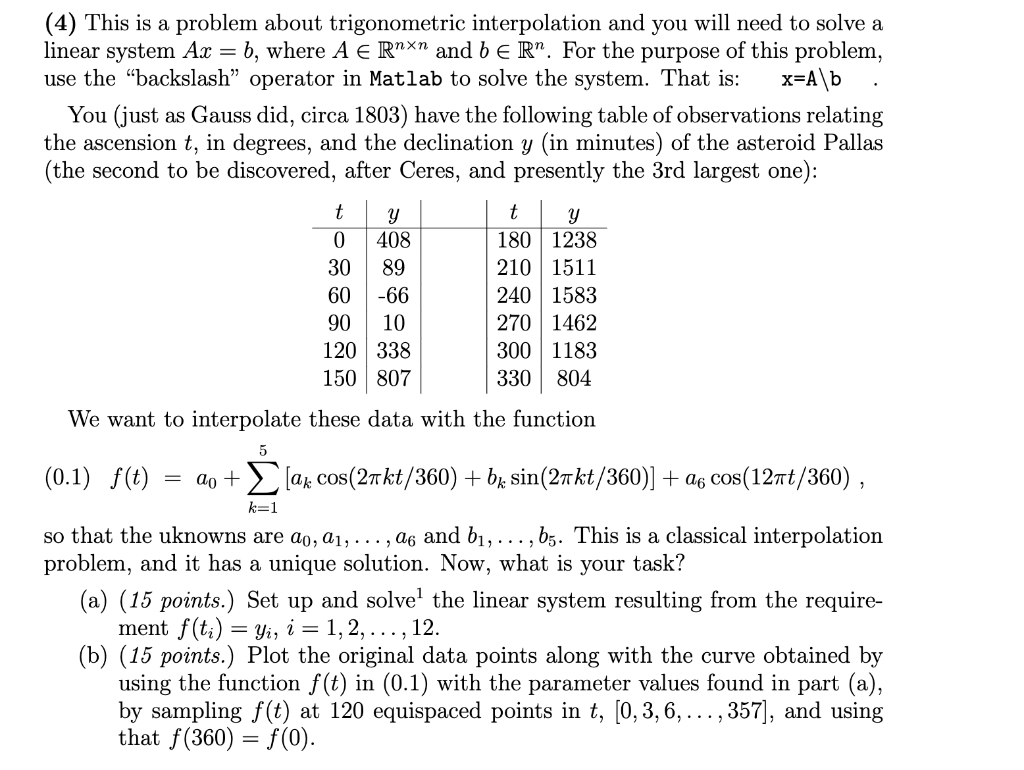# Question Use Matlab (4) This is a problem about trigonometric interpolation and you will need to solve a linear system $$A x=b$$, where $$A \in \mathbb{R}^{n \times n}$$ and $$b \in \mathbb{R}^{n}$$. For the purpose of this problem, use the "backslash" operator in Matlab to solve the system. That is: $$\quad \mathrm{x}=\mathrm{A} \backslash \mathrm{b}$$. You (just as Gauss did, circa 1803) have the following table of observations relating the ascension $$t$$, in degrees, and the declination $$y$$ (in minutes) of the asteroid Pallas (the second to be discovered, after Ceres, and presently the 3rd largest one): We want to interpolate these data with the function (0.1) $$f(t)=a_{0}+\sum_{k=1}^{5}\left[a_{k} \cos (2 \pi k t / 360)+b_{k} \sin (2 \pi k t / 360)\right]+a_{6} \cos (12 \pi t / 360)$$, so that the uknowns are $$a_{0}, a_{1}, \ldots, a_{6}$$ and $$b_{1}, \ldots, b_{5}$$. This is a classical interpolation problem, and it has a unique solution. Now, what is your task? (a) (15 points.) Set up and solve $${ }^{1}$$ the linear system resulting from the requirement $$f\left(t_{i}\right)=y_{i}, i=1,2, \ldots, 12$$. (b) (15 points.) Plot the original data points along with the curve obtained by using the function $$f(t)$$ in (0.1) with the parameter values found in part (a), by sampling $$f(t)$$ at 120 equispaced points in $$t,[0,3,6, \ldots, 357]$$, and using that $$f(360)=f(0)$$.Transcribed Image Text: (4) This is a problem about trigonometric interpolation and you will need to solve a linear system $$A x=b$$, where $$A \in \mathbb{R}^{n \times n}$$ and $$b \in \mathbb{R}^{n}$$. For the purpose of this problem, use the "backslash" operator in Matlab to solve the system. That is: $$\quad \mathrm{x}=\mathrm{A} \backslash \mathrm{b}$$. You (just as Gauss did, circa 1803) have the following table of observations relating the ascension $$t$$, in degrees, and the declination $$y$$ (in minutes) of the asteroid Pallas (the second to be discovered, after Ceres, and presently the 3rd largest one): We want to interpolate these data with the function (0.1) $$f(t)=a_{0}+\sum_{k=1}^{5}\left[a_{k} \cos (2 \pi k t / 360)+b_{k} \sin (2 \pi k t / 360)\right]+a_{6} \cos (12 \pi t / 360)$$, so that the uknowns are $$a_{0}, a_{1}, \ldots, a_{6}$$ and $$b_{1}, \ldots, b_{5}$$. This is a classical interpolation problem, and it has a unique solution. Now, what is your task? (a) (15 points.) Set up and solve $${ }^{1}$$ the linear system resulting from the requirement $$f\left(t_{i}\right)=y_{i}, i=1,2, \ldots, 12$$. (b) (15 points.) Plot the original data points along with the curve obtained by using the function $$f(t)$$ in (0.1) with the parameter values found in part (a), by sampling $$f(t)$$ at 120 equispaced points in $$t,[0,3,6, \ldots, 357]$$, and using that $$f(360)=f(0)$$.
Transcribed Image Text: (4) This is a problem about trigonometric interpolation and you will need to solve a linear system $$A x=b$$, where $$A \in \mathbb{R}^{n \times n}$$ and $$b \in \mathbb{R}^{n}$$. For the purpose of this problem, use the "backslash" operator in Matlab to solve the system. That is: $$\quad \mathrm{x}=\mathrm{A} \backslash \mathrm{b}$$. You (just as Gauss did, circa 1803) have the following table of observations relating the ascension $$t$$, in degrees, and the declination $$y$$ (in minutes) of the asteroid Pallas (the second to be discovered, after Ceres, and presently the 3rd largest one): We want to interpolate these data with the function (0.1) $$f(t)=a_{0}+\sum_{k=1}^{5}\left[a_{k} \cos (2 \pi k t / 360)+b_{k} \sin (2 \pi k t / 360)\right]+a_{6} \cos (12 \pi t / 360)$$, so that the uknowns are $$a_{0}, a_{1}, \ldots, a_{6}$$ and $$b_{1}, \ldots, b_{5}$$. This is a classical interpolation problem, and it has a unique solution. Now, what is your task? (a) (15 points.) Set up and solve $${ }^{1}$$ the linear system resulting from the requirement $$f\left(t_{i}\right)=y_{i}, i=1,2, \ldots, 12$$. (b) (15 points.) Plot the original data points along with the curve obtained by using the function $$f(t)$$ in (0.1) with the parameter values found in part (a), by sampling $$f(t)$$ at 120 equispaced points in $$t,[0,3,6, \ldots, 357]$$, and using that $$f(360)=f(0)$$.&#12304;General guidance&#12305;The answer provided below has been developed in a clear step by step manner.Step1/2Here's the MATLAB code for parts (a) and (b) separately:Part (a)% Given observationst = [0,30,60,90,120,150,180,210,240,270,300,330];y = [408,89,-66,10,338,807,1238,1511,1583,1462,1183,804];% Construct matrix An = length(t); % Number of observationsA = zeros(n, 12); % Initialize matrix AA(:, 1) = 1; % Set first column of A to 1for k = 1:5 A(:, 2*k) = cos(2*pi*k*t/360); A(:, 2*k+1) = sin(2*pi*k*t/360);endA(:, 12) = cos(12*pi*t/360);% Solve the linear system Ax = yx = A \ y';% Display the solutiondisp('Coefficients:');disp(x'); The code above corresponds to part (a) of the problem, where we need to set up and solve the linear system $$\mathrm{{A}{x}={y}}$$ to find the coefficients $$\mathrm{{a}_{{0}},{a}_{{1}},\ldots,{a}_{{6}}}$$ and $$\mathrm{{b}_{{1}},\ldots,{b}_{{5}}}$$ that interpolate the given observations. Here's an explanation of the code:We first define the given observations as two vectors, t and y.We then initialize the matrix A with zeros, and set the first column to 1 (for the constant term in the trigonometric function).We then loop over the indices k from 1 to 5, and compute the columns of A corresponding to the cosine and sine terms of the trigonometric function. We use the cos and sin functions, along with the values in the t vector, to compute these columns.Finally, we compute the column of A corresponding to the cosine term with frequency 12, and solve the linear system using the backslash operator in Matlab (x = A \ y'). The resulting vector x contains the coefficients of the trigonometric function that interpolate the observ ... See the full answer